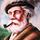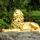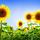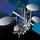## Social Question#### Can you find the next step in the solution to this math problem?

Asked by LostInParadise (30429) 2 months ago
6 responses
“Great Question” (1)

I thought of this problem while I was lying in bed. Zaku will no doubt complain about its relevance, but I thought it is a more interesting high school level math problem than the usual fare.

Find x and y such that x2 + y2 = 1,000,000. Note that for x = 8 and y = 6, x2 + y2 = 100. Do you see how to use that to find a simple solution? You can think of the (x, y) values as points in the plane.Follow this questionSend to a friend!Topics: ,
Observing members: 0Composing members: 0Geometrically the puzzle is equivalent to a ¾/5 triangle. If the square of the longest side is one million units the longest side must be 1,000 units in length that is 200 times 5. The other sides must then be 200 times 3 and 200 times 4, that is 600 and 800 respectively.

flutherother (33405)“Great Answer” (3)(Oh, you seem to mean x squared plus y squared.)

There are an infinite number of solutions for x and y.

Geometrically, your equation is the equation of a circle with radius 1000.

Any coordinate on that circle is a valid solution to the equation.

Zaku (28832)“Great Answer” (3)x is 1,000 and y is 1? Lol. I’m going to assume that’s not what you are looking for.

x is 800 and y is 600. It’s just a matter of the zeros being added to the right. Since I knew 1,000 times itself is a million, I knew to try 800 and 600 to get the correct amount of zeros.

JLeslie (63408)“Great Answer” (1)That technique gives whole numbers for all powers of 10 with an even numbered exponent. There is a similar technique for odd exponents. Can anybody find whole numbers x and y such that (x)2+(y)2=100,000.

It helps to recast the original method: 1,000,000 = 106 = 104×102 = 104(82+62) = (82×104)+ 62×104)=(8×102)2+(6×102)2=8002+6002.

In the case of 100,000=(10)5, we would start out with 100,000=(10) 5=10^4×10 = ...

LostInParadise (30429)“Great Answer” (1)100,000 = 3002+1002. For odd powers of 10 you use the fact that 10=32+12
100,000 = 105=104×10 = 104(32+1)= 32×104+12×104 = (3×102)2 + (102)2 = 3002 + 1002

LostInParadise (30429)“Great Answer” (0)You want to go from 1e2 to 1e6. That is a factor of 1e4. The quantities are squared, so you use a factor of 1e2 to get 1e4. Thus, multiply 8 by 100 and 6 by 100, and you will get your 1e6.

RocketGuy (14330)“Great Answer” (1)